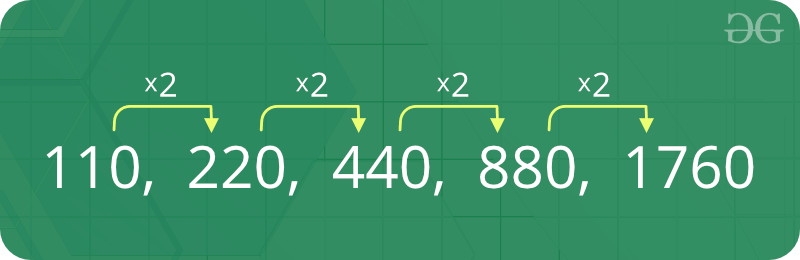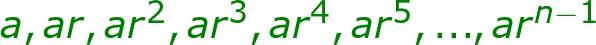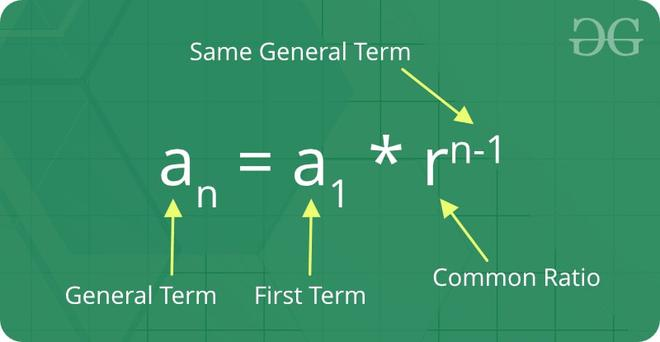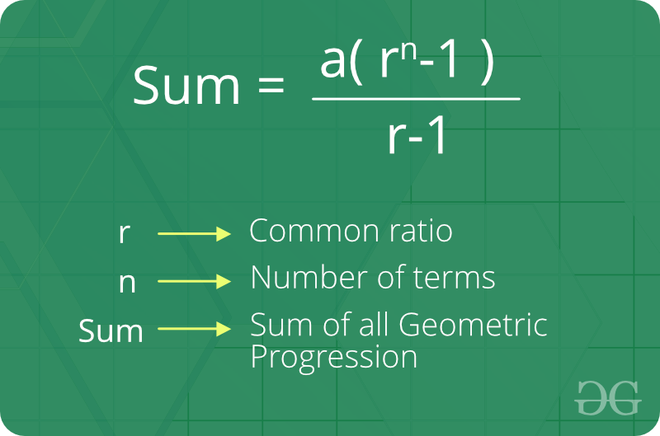Open in App
Not now

# Geometric Progression

• Last Updated : 01 Sep, 2022

A sequence of numbers is called a Geometric progression if the ratio of any two consecutive terms is always the same.In simple terms, A geometric series is a list of numbers where each number, or term, is found by multiplying the previous term by a common ratio r. The general form of Geometric Progression is:GP-series

Where,
a = First term
r = common ratio
arn-1 = nth term

Example:

The sequence 2, 4, 8, 16 is a GP because ratio of any two consecutive terms in the series (common difference) is same (4 / 2 = 8 / 4 = 16 / 8 = 2).

### The geometric progression is of two types:

1. Finite geometric progression
2. Infinite geometric progression.

### 1. Finite geometric progression

In finite geometric progression contains a finite number of terms. The last term is always defined in this type of progression.

Example:

The sequence 1/2,1/4,1/8,1/16,…,1/32768 is a finite geometric series where the first term is 1/2 and the last term is 1/32768.

### 2. Infinite geometric progression

Infinite geometric progression contains an infinite number of terms.  The last term is not defined in this type of progression.

### Example:

Sequence 3, 9, 27, 81, … is an infinite series where the first term is 3 but the last term is not defined.

1. Initial term: In a geometric progression, the first number is called the initial term.
2. Common ratio: The ratio between a term in the sequence and the term before it is called the “common ratio.”
3. The behavior of a geometric sequence depends on the value of the common ratio. If the common ratio is:
• Positive, the terms will all be the same sign as the initial term.
• Negative, the terms will alternate between positive and negative.
• Greater than 1, there will be exponential growth towards positive or negative infinity (depending on the sign of the initial term).
• 1, the progression is a constant sequence.
• Between -1 and 1 but not zero, there will be exponential decay towards zero.
• -1, the progression is an alternating sequence.
• Less than -1, for the absolute values there is exponential growth towards (unsigned) infinity, due to the alternating sign.

### The formula for the nth term of a Geometric Progression:

If ‘a1′ is the first term and ‘r’ is the common ratio. Thus, the explicit formula for nth term of finite GP series:Nth term of a Geometric Progression

The formula for the sum of the nth term of Geometric Progression:Sum of the Nth term of Geometric Progression

How do we check whether a series is a Geometric progression or not?

The property of the GP series is that the ratio of the consecutive terms is same.

Approach:

1. First calculate the common ratio r by arr / arr
2. Iterate over an array and calculate the ratio of the consecutive terms.
3. Check if the calculated ratio is not equal to the common ratio r
• Return false
4. After traversal, if the calculated ratio is equal to the common ratio r every time
• Return true

Below is the implementation of the above approach:

## C++

 `// C++ program to check if a given array``// can form geometric progression` `#include ` `using` `namespace` `std;` `bool` `is_geometric(``int` `arr[], ``int` `n)` `{``    ``if` `(n == 1)``        ``return` `true``;` `    ``// Calculate ratio``    ``int` `ratio = arr / (arr);` `    ``// Check the ratio of the remaining``    ``for` `(``int` `i = 1; i < n; i++) {``        ``if` `((arr[i] / (arr[i - 1])) != ratio) {``            ``return` `false``;``        ``}``    ``}``    ``return` `true``;``}` `// Driven Program``int` `main()``{``    ``int` `arr[] = { 2, 6, 18, 54 };``    ``int` `n = ``sizeof``(arr) / ``sizeof``(arr);` `    ``(is_geometric(arr, n)) ? (cout << ``"True"` `<< endl)``                           ``: (cout << ``"False"` `<< endl);` `    ``return` `0;``}`

## Java

 `// Java program to check if a given array``// can form geometric progression``import` `java.util.Arrays;` `class` `GFG {` `    ``// function to check series is``    ``// geometric progression or not``    ``static` `boolean` `is_geometric(``int` `arr[], ``int` `n)``    ``{``        ``if` `(n == ``1``)``            ``return` `true``;` `        ``// Calculate ratio``        ``int` `ratio = arr[``1``] / (arr[``0``]);` `        ``// Check the ratio of the remaining``        ``for` `(``int` `i = ``1``; i < n; i++) {``            ``if` `((arr[i] / (arr[i - ``1``])) != ratio) {``                ``return` `false``;``            ``}``        ``}``        ``return` `true``;``    ``}` `    ``// driver code``    ``public` `static` `void` `main(String[] args)``    ``{``        ``int` `arr[] = { ``2``, ``6``, ``18``, ``54` `};``        ``int` `n = arr.length;` `        ``if` `(is_geometric(arr, n))``            ``System.out.println(``"True"``);``        ``else``            ``System.out.println(``"False"``);``    ``}``}`

## Python3

 `def` `is_geometric(li):``    ``if` `len``(li) <``=` `1``:``        ``return` `True``        ` `    ``# Calculate ratio``    ``ratio ``=` `li[``1``]``/``float``(li[``0``])``    ` `    ``# Check the ratio of the remaining``    ``for` `i ``in` `range``(``1``, ``len``(li)):``        ``if` `li[i]``/``float``(li[i``-``1``]) !``=` `ratio:``            ``return` `False``    ``return` `True` `print``(is_geometric([``2``, ``6``, ``18``, ``54``]))`

## C#

 `// C# program to check if a given array``// can form geometric progression``using` `System;` `class` `Geeks {` `    ``static` `bool` `is_geometric(``int``[] arr, ``int` `n)``    ``{``        ``if` `(n == 1)``            ``return` `true``;` `        ``// Calculate ratio``        ``int` `ratio = arr / (arr);` `        ``// Check the ratio of the remaining``        ``for` `(``int` `i = 1; i < n; i++) {``            ``if` `((arr[i] / (arr[i - 1])) != ratio) {``                ``return` `false``;``            ``}``        ``}``        ``return` `true``;``    ``}` `    ``// Driven Program``    ``public` `static` `void` `Main(String[] args)``    ``{``        ``int``[] arr = ``new` `int``[] { 2, 6, 18, 54 };``        ``int` `n = arr.Length;` `        ``if` `(is_geometric(arr, n))``            ``Console.WriteLine(``"True"``);``        ``else``            ``Console.WriteLine(``"False"``);``    ``}``}`

## PHP

 ``

## Javascript

 ``

Output

`True`

Time Complexity: O(n), Where n is the length of the given array.

Auxiliary Space: O(1)

Basic Program related to Geometric Progression

More problems related to Geometric Progression

Recent Articles on Geometric Progression!

My Personal Notes arrow_drop_up